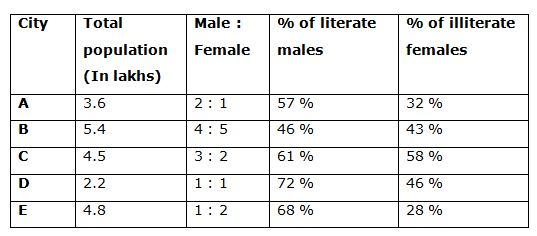# CWC/FCI Prelims 2019 – Quantitative Aptitude Questions (Day-07)

Dear Readers, Exam Race for the Year 2019 has already started, To enrich your preparation here we are providing new series of Practice Questions on Quantitative Aptitude – Section for CWC/FCI Exam. Aspirants, practice these questions on a regular basis to improve your score in aptitude section. Start your effective preparation from the right beginning to get success in upcoming CWC/FCI Exam.

[WpProQuiz 5457]

Directions (Q. 1 – 5): What approximate value should come in place of (?) in the following questions?

1) 49.21 × 19.89 – 24.98 × 14.12 = (36 +?) × 9

a) 22

b) 46

c) 58

d) 34

e) 70

2) 35 % of 1599 + 29 % of 4501 =? × 41 + 468 + 773.89 – 199.78

a) 24

b) 20

c) 32

d) 45

e) 10

3) (63.89)2 × (64.12)1/3 × (25.99)2 ÷ (211 × (12.77)2) = 2x

a) 6

b) 7

c) 5

d) 8

e) 4

4) (1782 ÷ 11.98) ÷ 2.92 × 35.89 + 25 % of 541 – 67 % of 1299 = ?

a) 1046

b) 1153

c) 982

d) 1268

e) 1375

5) 5 (7/8) + 9 (3/16) – 7 (5/9) =? + 3 (2/3)

a) 5

b) 3

c) 7

d) 9

e) 6

Directions (6 – 10): Study the following information carefully and answer the questions given below.

The following table shows the total population of 5 different cities and the ratio of male to that of female and the percentage of literate males and the percentage of illiterate females among them.6) Find the total literate population in city A

a) 198500

b) 185600

c) 218400

d) 237800

e) 274500

7) The total male population in City B and C together is approximately what percentage more/less than the total female population in City A and D together?

a) 122 % more

b) 108 % less

c) 108 % more

d) 122 % less

e) 85 % more

8) Find the ratio between the literate males in City B to that of literate females in City E

a) 25: 48

b) 23: 48

c) 48: 29

d) 38: 23

e) None of these

9) Find the average number of female population in all five cities?

a) 195000

b) 178000

c) 206000

d) 232000

e) 164000

10) In which city has less number of illiterate female population?

a) City A

b) City C

c) City B

d) City D

e) City E

Directions (Q. 1-5):

49 × 20 – 25 × 14 = (36 + x) × 9

980 – 350 = 324 + 9x

9x = 980 – 350 – 324

9x = 306

X = 34

(35/100)*1600 + (29/100)*4500 = 41x + 468 + 774 – 200

560 + 1305 – 468 – 774 + 200 = 41x

823 = 41x

X = 820/41 = 20

(64 × 64 × 4 × 26 × 26) / (211 × 13 × 13) = 2x

(26 × 26 × 24)/ 211 = 2x

26 + 6 + 4 – 11 = 2x

25 = 2x

X = 5

(1782/12) × (1/3) × 36 + (25/100) × 540 – (67/100) × 1300 = x

1782 + 135 – 871 = x

X = 1046

5 (7/8) + 9 (3/16) – 7 (5/9) =? + 3 (2/3)

6 + 9 – 8 – 4 = x

X = 3

Directions (Q. 6-10):

The total literate population in city A,

= > 360000*(2/3)*(57/100) + 360000*(1/3)*(68/100)

= > 136800 + 81600 = 218400

The total male population in City B and C together,

= > [(5.4/9)*4 + (4.5/5)*3] lakhs

= > 2.4 + 2.7 = 5.1 lakhs

The total female population in City A and D together,

= > [(3.6/3)*1 + (2.2/2)*1] lakhs

= > 1.2 + 1.1 = 2.3 lakhs

Required % = [(5.1 – 2.3)/2.3]*100 = 121.739 % = 122 % more

Total male population in city B = (5.4/9)*4 = 2.4 lakhs

The total number of literate males in City B,

= > 240000*(46/100) = 110400

Total female population in city E = (4.8/3)*2 = 3.2 lakhs

The total number of literate females in City E,

= > 320000*(72/100) = 230400

Required ratio = 110400: 230400 = 1104: 2304 = 23: 48

Total number of female population in all five cities is,

= > (3.6/3)*1 + (5.4/9)*5 + (4.5/5)*2 + (2.2/2)*1 + (4.8/3)*2

= > 1.2 + 3 + 1.8 + 1.1 + 3.2 = 10.3 lakhs

Required average = 1030000/5 = 206000

City A = 120000*(32/100) = 38400

City B = 300000*(43/100) = 129000

City C = 180000*(58/100) = 104400

City D = 110000*(46/100) = 50600

City E = 320000*(28/100) = 89600

So, City A has less number of illiterate female population.